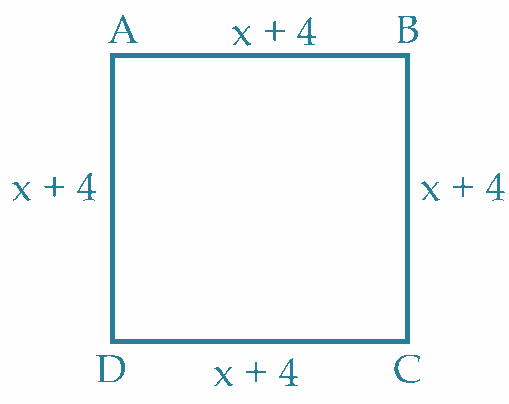A garden is in the shape of a square as shown adjacent. The area of the square ABCD is 289 sq. meters with each side (x+4) m. Based on above information, the value of x is

# A garden is in the shape of a square as shown adjacent. The area of the square ABCD is 289 sq. meters with each side (x+4) m. Based on above information, the value of x is1. A
x = 12
2. B
x = 11
3. C
x = 13
4. D
x = 14

Fill Out the Form for Expert Academic Guidance!l

+91

Live ClassesBooksTest SeriesSelf Learning

Verify OTP Code (required)

### Solution:

Given that the area of the square ABCD is 289 sq. meters with each side (x+4) m.
We know that, Area of a square = .
Therefore, Area of the square = .
Already given, area of square is 289.
So,

= 17
x = 17 - 4
x = 13
As a result, the needed value of x = 13.
The correct option is (3).

## Related content

 Difference Between Mass and Weight Differences & Comparisons Articles in Physics Important Topic of Physics: Reynolds Number Distance Speed Time Formula Refractive Index Formula Mass Formula Electric Current Formula Electric Power Formula Resistivity Formula Weight Formula+91

Live ClassesBooksTest SeriesSelf Learning

Verify OTP Code (required)